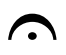Musical Symbols / Notation: the Classic FM guide

21 October 2014, 17:18 | Updated: 6 January 2017, 14:45

Your comprehensive guide to musical notation and symbols.

Rest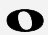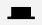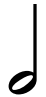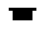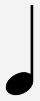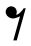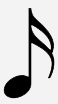= Semi-quaver / Sixteenth note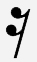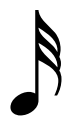= Demi-semi-quaver / Thirty-second note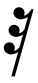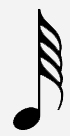= Hemi-demi-semi-quaver / Sixty-fourth note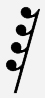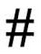= Sharp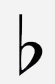Dynamics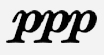= Piano pianissimo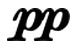= Pianissimo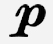= Piano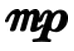= Mezzo-piano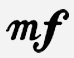= Mezzo-forte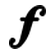= Forte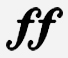= Fortissimo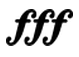= Forte fortissimo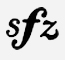= Sforzando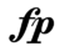Miscellaneous Symbols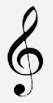= Treble clef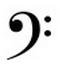= Bass clef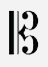= Tenor clef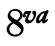= Ottava alta (an octave above)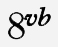= Ottava bassa (an octave below)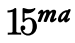= Two octaves above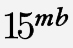=  Two octaves below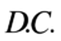= Da capo (from the beginning)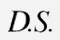= Dal segno (from the sign)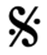= Segno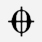= Coda (go to the coda)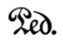= Sustain pedal down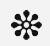= Release pedal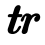= Trill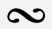= Turn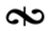= Inverted-turn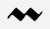= Mordent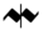= Inverted mordent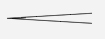= Crescendo (hairpin)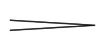= Diminuendo / decrescendo (hairpin)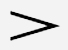= Accent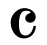= Common time (4/4)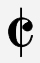= Alla breve / Cut time (2/2)